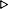ToC DocOverview CGDoc RelNotes Index PermutedIndex
Allegro CLANSI Common Lisp3 Evaluation and Compilation3.8 Dictionary Evaluation and Compilation

 3.8.9 define-compiler-macro Macro

Syntax:
define-compiler-macro name lambda-list [[{declaration}* \$|\$ documentation]] {form}*name

Arguments and Values:
name - a function name.

lambda-list - a macro lambda list.

declaration - a declare expression; not evaluated.

documentation - a string; not evaluated.

form - a form.

Description:

This is the normal mechanism for defining a compiler macro function. Its manner of definition is the same as for defmacro; the only differences are:

Examples:
``` (defun square (x) (expt x 2))SQUARE
(define-compiler-macro square (&whole form arg)
(if (atom arg)
`(expt ,arg 2)
(case (car arg)
(square (if (= (length arg) 2)
`(expt ,(nth 1 arg) 4)
form))
(expt   (if (= (length arg) 3)
(if (numberp (nth 2 arg))
`(expt ,(nth 1 arg) ,(* 2 (nth 2 arg)))
`(expt ,(nth 1 arg) (* 2 ,(nth 2 arg))))
form))
(otherwise `(expt ,arg 2)))))SQUARE
(square (square 3))81
(macroexpand '(square x))(SQUARE X), false
(funcall (compiler-macro-function 'square) '(square x) nil)(EXPT X 2)
(funcall (compiler-macro-function 'square) '(square (square x)) nil)(EXPT X 4)
(funcall (compiler-macro-function 'square) '(funcall #'square x) nil)(EXPT X 2)

(defun distance-positional (x1 y1 x2 y2)
(sqrt (+ (expt (- x2 x1) 2) (expt (- y2 y1) 2))))DISTANCE-POSITIONAL
(defun distance (&key (x1 0) (y1 0) (x2 x1) (y2 y1))
(distance-positional x1 y1 x2 y2))DISTANCE
(define-compiler-macro distance (&whole form
&rest key-value-pairs
&key (x1 0  x1-p)
(y1 0  y1-p)
(x2 x1 x2-p)
(y2 y1 y2-p)
&allow-other-keys
&environment env)
(flet ((key (n) (nth (* n 2) key-value-pairs))
(arg (n) (nth (1+ (* n 2)) key-value-pairs))
(simplep (x)
(let ((expanded-x (macroexpand x env)))
(or (constantp expanded-x env)
(symbolp expanded-x)))))
(let ((n (/ (length key-value-pairs) 2)))
(multiple-value-bind (x1s y1s x2s y2s others)
(loop for (key) on key-value-pairs by #'cddr
count (eq key ':x1) into x1s
count (eq key ':y1) into y1s
count (eq key ':x2) into x2s
count (eq key ':y1) into y2s
count (not (member key '(:x1 :x2 :y1 :y2)))
into others
finally (return (values x1s y1s x2s y2s others)))
(cond ((and (= n 4)
(eq (key 0) :x1)
(eq (key 1) :y1)
(eq (key 2) :x2)
(eq (key 3) :y2))
`(distance-positional ,x1 ,y1 ,x2 ,y2))
((and (if x1-p (and (= x1s 1) (simplep x1)) t)
(if y1-p (and (= y1s 1) (simplep y1)) t)
(if x2-p (and (= x2s 1) (simplep x2)) t)
(if y2-p (and (= y2s 1) (simplep y2)) t)
(zerop others))
`(distance-positional ,x1 ,y1 ,x2 ,y2))
((and (< x1s 2) (< y1s 2) (< x2s 2) (< y2s 2)
(zerop others))
(let ((temps (loop repeat n collect (gensym))))
`(let ,(loop for i below n
collect (list (nth i temps) (arg i)))
(distance
,@(loop for i below n
append (list (key i) (nth i temps)))))))
(t form))))))DISTANCE
(dolist (form
'((distance :x1 (setq x 7) :x2 (decf x) :y1 (decf x) :y2 (decf x))
(distance :x1 (setq x 7) :y1 (decf x) :x2 (decf x) :y2 (decf x))
(distance :x1 (setq x 7) :y1 (incf x))
(distance :x1 (setq x 7) :y1 (incf x) :x1 (incf x))
(distance :x1 a1 :y1 b1 :x2 a2 :y2 b2)
(distance :x1 a1 :x2 a2 :y1 b1 :y2 b2)
(distance :x1 a1 :y1 b1 :z1 c1 :x2 a2 :y2 b2 :z2 c2)))
(print (funcall (compiler-macro-function 'distance) form nil)))(LET ((#:G6558 (SETQ X 7))(#:G6559 (DECF X))(#:G6560 (DECF X))(#:G6561 (DECF X)))(DISTANCE :X1 #:G6558 :X2 #:G6559 :Y1 #:G6560 :Y2 #:G6561))(DISTANCE-POSITIONAL (SETQ X 7) (DECF X) (DECF X) (DECF X))(LET ((#:G6567 (SETQ X 7))(#:G6568 (INCF X)))(DISTANCE :X1 #:G6567 :Y1 #:G6568))(DISTANCE :X1 (SETQ X 7) :Y1 (INCF X) :X1 (INCF X))(DISTANCE-POSITIONAL A1 B1 A2 B2)(DISTANCE-POSITIONAL A1 B1 A2 B2)(DISTANCE :X1 A1 :Y1 B1 :Z1 C1 :X2 A2 :Y2 B2 :Z2 C2)NIL
```